## Symmetries: Why Physicists Care

Classification is key to science in many ways; the classification of particles according to various properties - spin, mass, charge, ... - is one of the key concepts in physics. But why should such a classification exist and be useful, and how can we understand the one we see?

The key idea is to link these classifications with the concept of a symmetry. One can use Noether's theorem to understand how a symmetry gives rise to a conserved quantity; in contrast, one uses representation theory to understand how expected symmetries of the real world can be manifested by particles. The former is a very direct way to obtain expressions for conserved quantities corresponding to the properties above; the latter gives a fundamental insight into what is happening for each individual particle species, and is needed to correctly, say, obtain the possible spins of particles in our spacetime.

### What is a symmetry?

We'll suppose our theory is defined by a bunch of fields (each of which will usually correspond to either one particle, or some fairly small number of related particles) which we shall lump together symbolically into the vector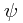, and a Lagrangian density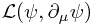. We emphasize 'density' here to clarify that the Lagrangian is something different -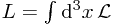. Of course, if you are familiar with the principles of special (or general) relativity, you might be a bit upset at the use of the integral over space only, since that is not covariant. In that case, you would be more happy with the definition of the action S, a functional depending on the particular set of (classical) fields given: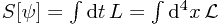Now we can define a symmetry straightforwardly: it is a map taking the function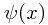to a new function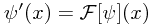where the curly F represents the symmetry, which obeys the requirement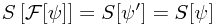for all fields. Formally, such an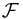is a symmetry of the action.

Note: If you're unhappy with the notion of a Lagrangian... you need to become happy with it! If you prefer, though, conceptually you can just think of something more like the Hamiltonian, or the energy of the system, in place of the Lagrangian. As for a concrete example, a massless scalar field would classically be a scalar function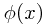with Lagrangian density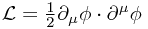(in the +--- sign convention beloved of most particle physicists).

Examples: One might define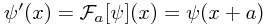for some constant vector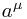. Then the symmetry is called a translational symmetry. This is a symmetry of any complete flat-space system which treats all points equally. Note that this is (if I understand the terminology correctly, though I have never cared to try!) an active transformation, because we are currently thinking of a prescriptionwhich takes one stateand gives us a new one,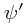defined on the same space. However, we are clearly free to think of this differently, and think of the related passive transformation, in which we actually just change the coordinate system, so that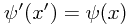where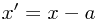, and the two functions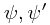are the same function, evaluated in different coordinate systems.

This particular alternative view does not hold for different symmetries, however; it might be that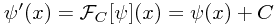is a symmetry for some constant number C, as for the free massless scalar field, for example. This could be a very simple gauge symmetry, and cannot be achieved by a change of coordinate. For this reason, I prefer to think in terms of the 'active' viewpoint, where a symmetry takes your old field and gives you a new one. Note, however, that gauge transformations (relating physically equivalent states) are very different to symmetries arising from coordinate transformations. [Mention the Coleman-Mandula theorem and supersymmetry.]

### What does this mean?

A symmetry of the action should correspond to a symmetry observed by someone conducting experiments. This is because (most obviously via Feynman path integrals) the action alone determines the physical rules obeyed by the system.

As mentioned above, Noether's theorem now gives an expression for a conserved current, with the property that the spatial integral of the 0 component is constant in time classically; in the quantum context, one finds an integral operator commuting with the Hamiltonian - thus one can simultaneously diagonalize both, and then the value of the integral operator is constant for an eigenbasis element. Instead, let us consider the actual behaviour of classical solutions under these transformations.

Note: A natural question to ask is "Why do we focus on classical solutions?" The most useful answer is to consider the procedure of quantization; for example, in canonical quantization of a free field, one expands the field operator in terms of a basis of classical solutions multiplied by related creation/annihilation operators, each of which corresponds to a particular excitation, and ultimately particle, one hopes. Thus classifying classical solutions nicely should correspond to classifying particles nicely.

The key assumption we will use in our classification is that the free particle theory, with linear equations of motion, is a good approximation to our theory, in some sense; most naïvely, one wants non-linear terms to have small coefficients. (Lots of subtleties come in - for example, renormalization means that these other terms can effectively correct the other terms non-trivially.) This is because we want to think only about these simple free field solutions.

### Reversing the logic

We now want to understand not how nice symmetries of special Lagrangian densities lead to nice observable symmetries, but what the nature of particles must be in order to respect the symmetries inherent in three-dimensional space.

What do I mean? Let us assume that, to conform with our physical expectations, a system is translationally symmetric in the sense defined above. Then we expect that each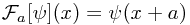is a symmetry of the system, whatever the unknownare. The key point here is that, supposing we have some basis for the classical solutions - this being a linear space, crucially - the right-hand side lies within the same space.

Now suppose that we could diagonalize this operatorfor all a - note that all the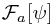obviously commute, so this is reasonable. What do I mean by this? I want to find a collection of functions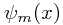for some label m with the property that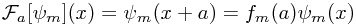for some eigenvalues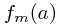. In general,represents a column vector of fields, and we will allow the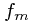to be matrices in this case. Were we to find such a 'nice' basis, we can use our knowledge of the behaviour of transformations to deduce information about thematrices.

We know that composing two translations is just the same as doing both together: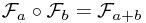. This means that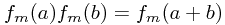- this is quite a restriction on the possible ways that the f matrices can depend on the translation vector! In fact, if we only had a single field, then we could deduce (take logs) that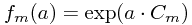for some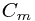. (We could drop the 'for all a' bit above, and just consider translations by a. However, we would then deduce that it worked for all integer multiples of a, which is less strong - see below.)

What can thebe? Remembering the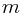labels are arbitrary, we note that the dependence onisn't interesting. If we assume that the action is quadratic in the fields, though, we note that there is no wayhas non-zero real part, as this would lead to unbounded contributions to some carefully chosen integrals, violating the condition that this is a symmetry. Hence without loss of generality,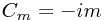, andis essentially the momentum of the corresponding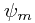. (See the next section for clarity.)

This is a bit unsatisfactory; we've posited the existence of some magical eigenbasis solving infinitely many different eigenvalue problems (the 'for all a' bit above) even if the terms did commute, and then dismissed the multi-field case. We will attempt to improve the former case first, and then see below how representation theory answers questions like that arising in arbitrary dimensions.

### Infinitesimal approach

We definitely expect arbitrarily small translations to be symmetries of the system. What form do these take?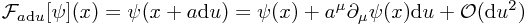Hence, dropping second order terms, we have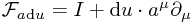which is a nice local operator. Suppose we diagonalize this operator for some a. Then we have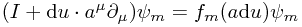or, separating out 0th and 1st order terms,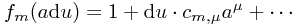for some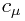such that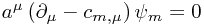Now note that if we solve the eigenvalue problem for just one such a, we obtain a solution for all multiples of a. Hence focusing on just one direction at a time, say a coordinate direction, we note that diagonalizing four commuting operators is sufficient to diagonalize all infinitesimal transformations.

Now we are done! In the 1-field case, the 4-vector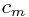characterizes each solution, and can be seen to give the momentum - we have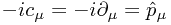.

### Outlook: Rotations and spin

Now we have seen how, in a very simple case, we can obtain a way of characterizing a basis of particle states using a symmetry. The key idea was looking for a basis which was transformed in a simple local way - essentially an eigenbasis of the symmetry - and investigating the behaviour of the 'eigenvalues', which can in general be constant matrices, under composition of symmetries. We have seen this only in a very simple case.

A much more rich topic is that associated with rotations. (In the following brief section, we will imply we are working with SO(3), but in fact - as outlined elsewhere on the site, one should in fact work with SU(2), as we will do in the following section.) Here, we have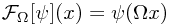where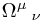is a rotation matrix. Now we have composition being the non-abelian matrix multiplication of the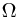matrices. This means, amongst other things, that the big 1D simplifications of the above case cannot work except in the most trivial case. Why? Suppose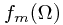is just a number, not a matrix. Then we have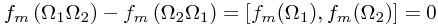but it is easy to show (a good exercise, involving choosing one matrix given the other is a rotation by θ about some axis, and deducing that rotating by θ has the same f as rotating by -θ, and since composition gives the identity,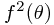= 1) that this means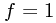is the only non-zero solution. (You might like to try this infinitesimally too!)

So we need to venture into understanding what possible interesting forms the matrixcan take whilst preserving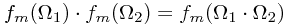## Representation Theory

Let us (in the light of the note above, which referred to this other article for justification) think about what possible matrices could obey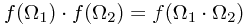and also, obviously,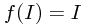for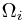in the group SU(2) of special unitary matrices, and I standing for two (in general of different size!) identity matrices. To get a grip on this, we make some definitions...